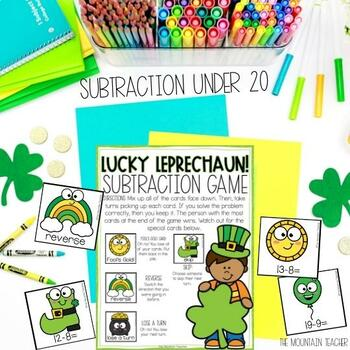# St. Patrick's Day Addition & Subtraction Activities - 4 March Math Games

Rated 5 out of 5, based on 2 reviews
2 Ratings;
K - 3rd
Subjects
Resource Type
Standards
Formats Included
• PDF
Pages
20 pages
Report this resource to TPT

#### What educators are saying

My students love these! They improve their math fact fluency after practicing with these every time they use them. Thank you!

### Description

Looking for some low prep but high engagement St. Patrick's Day addition and subtraction math games? Students can practice addition and subtraction facts to 20 DAILY with these 4 fun St. Patrick's Day addition and subtraction math fact fluency games. They won't even know they are learning!

Skills Practiced with St. Patrick's Day Addition and Subtraction Math Activities:

Students will practice their math fact fluency to 20 using addition and subtraction with these 4 fun St. Patrick's Day math games.

4 St. Patrick's Day Math Games Included:

• Addition to 20 Bump Game (good for partners or groups of 3)
• Subtraction to 20 Bump Game (good for partners or groups of 3)
• Lucky Leprechaun Addition to 20 Game (good for groups of 3+)
• Lucky Leprechaun Subtraction to 20 Game (good for groups of 3+)

Bump games are no prep, and the Lucky Leprechaun games need to be cut out and possibly laminated, based on preference.

When to use the St. Patrick's Day Math Games:

These St. Patrick's Day addition and subtraction math games are PERFECT for:

• Early Finishers
• Valentine's Day Math Centers
• Small Groups
• Whole Groups
• Valentine's Day Class Parties
• Fun Friday
• Snowy Days
• The Day Before Winter Break

& more

What Ages Can Use the St. Patrick's Day Addition & Subtraction Math Games?

The St. Patrick's Day math games are perfect for students in kindergarten, 1st, 2nd or 3rd grade. However, any student working on math fact fluency to 20 with addition and subtraction can use these.

What Supplies Will I Need for the St. Patrick's Day Math Games?

The Lucky Leprechaun game has everything students need.

For the BUMP games, students will need 2 dice and each student will need 8 game pieces. Game pieces can be any math manipulative, mini erasers, or small pieces of paper.

Total Pages
20 pages
Does not apply
Teaching Duration
1 month
Report this resource to TPT
Reported resources will be reviewed by our team. Report this resource to let us know if this resource violates TPT’s content guidelines.

### Standards

to see state-specific standards (only available in the US).
Represent addition and subtraction with objects, fingers, mental images, drawings, sounds (e.g., claps), acting out situations, verbal explanations, expressions, or equations.
Solve addition and subtraction word problems, and add and subtract within 10, e.g., by using objects or drawings to represent the problem.
Apply properties of operations as strategies to add and subtract. If 8 + 3 = 11 is known, then 3 + 8 = 11 is also known. (Commutative property of addition.) To add 2 + 6 + 4, the second two numbers can be added to make a ten, so 2 + 6 + 4 = 2 + 10 = 12. (Associative property of addition.)
Fluently add and subtract within 20 using mental strategies. By end of Grade 2, know from memory all sums of two one-digit numbers.